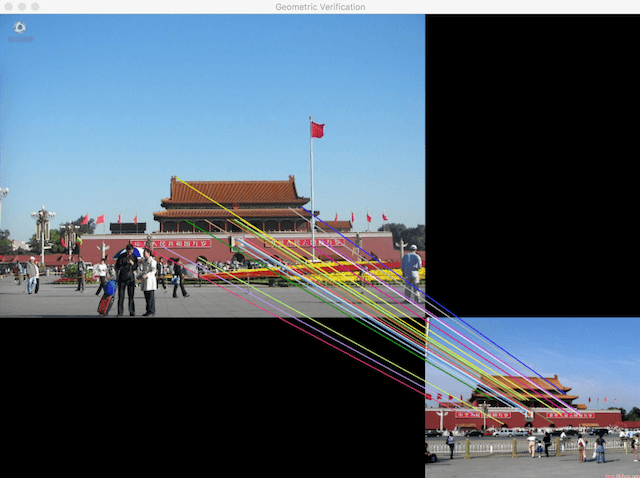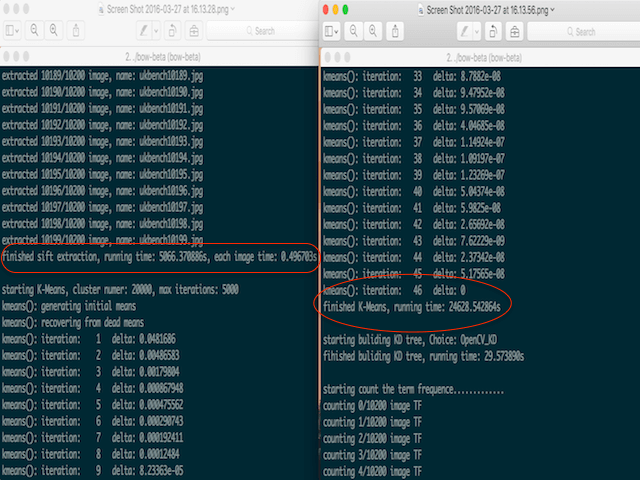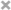## 选取合适的库

#### SIFT特征抽取提取选用哪个库？

• Lowe的SIFT，效果只提供SIFT的二进制可执行文件，弃用；
• Robwhess的OpenSIFT，开源，效果也还不错，需要一些别的依赖库，不再更新，弃用；
• OpenCV的SIFT，这个当然在使用上是最方便的，文档全，不依赖别的库，但SIFT的实现效果并不是很好，弃用；
• VLfeat里的SIFT，SIFT的实现效果是比较好的，缺点是c接口文档不怎么全，网上提供的资料也比较少，但多读读它的C源码，还是可以搞定的，而且在不用依赖其他的库，所以选择这个库来提取SIFT还是很好的，在实际提取的时候，我选用的是covdet函数来提取SIFT，它是一个功能更强大的提取co-variant特征提取器。## 词袋模型的类类型设计

class siftDesctor{
public:
siftDesctor(){};
std::string imageName;
std::vector<std::vector<float>> frame;
std::vector<std::vector<float>> desctor;
void covdet_keypoints_and_descriptors(cv::Mat &img, std::vector<std::vector<float>> &frames, std::vector<std::vector<float>> &desctor, bool rooSIFT, bool verbose);
std::vector<float> rootsift(std::vector<float> &dst);

void Serialize(std::ofstream &outfile) const {
std::string tmpImageName = imageName;
int strSize = (int)imageName.size();
outfile.write((char *)&strSize, sizeof(int));
outfile.write((char *)&tmpImageName, sizeof(char)*strSize); // 写入文件名

int descSize = (int)desctor.size();
outfile.write((char *)&descSize, sizeof(int));

// 写入sift特征
for(int i = 0; i < descSize; i++ ){
outfile.write((char *)&(desctor[i]), sizeof(float) * 128);
outfile.write((char *)&(frame[i]), sizeof(float) * 6);
}

}

static siftDesctor Deserialize(std::ifstream &ifs) {
siftDesctor siftDesc;
int strSize = 0;
siftDesc.imageName = "";
siftDesc.imageName.resize(strSize);

int descSize = 0;

// 读入sift特征和frame
for(int i = 0; i < descSize; i++ ){
std::vector<float> tmpDesc(128);
siftDesc.desctor.push_back(tmpDesc);

std::vector<float> tmpFrame(6);
siftDesc.frame.push_back(tmpFrame);
}

return siftDesc;
}

};


class bowModel {
public:
bowModel(){};
bowModel(int _numWords,std::vector<siftDesctor> _imgFeatures, std::vector<std::vector<int>> _words):numWords(_numWords),imgFeatures(_imgFeatures),words(_words){};

int numNeighbors = 1;
int numWords;
std::vector<siftDesctor> imgFeatures;
std::vector<std::vector<int>> words;
cv::Mat centroids_opencvMat;

cv::flann::Index opencv_buildKDTree(cv::Mat &centroids_opencvMat);

void Serialize(std::ofstream &outfile) const {
int imgFeatsSize = (int)imgFeatures.size();
outfile.write((char *)&imgFeatsSize, sizeof(int));
// 写入imgFeatures和words
for(int i = 0; i < imgFeatsSize; i++ ){
imgFeatures[i].Serialize(outfile);
outfile.write((char *)&(words[i]), sizeof(int) * imgFeatures[i].desctor.size());
}

}

static bowModel Deserialize(std::ifstream &ifs) {
bowModel BoW;
int imgFeatsSize;

BoW.words.resize(imgFeatsSize);

for (int i = 0; i < imgFeatsSize; i++) {
// 读入imgFeatures
auto siftDesc = siftDesctor::Deserialize(ifs);
BoW.imgFeatures.push_back(siftDesc);
// 读入words
BoW.words[i].resize(siftDesc.desctor.size());
}
return BoW;
}

};